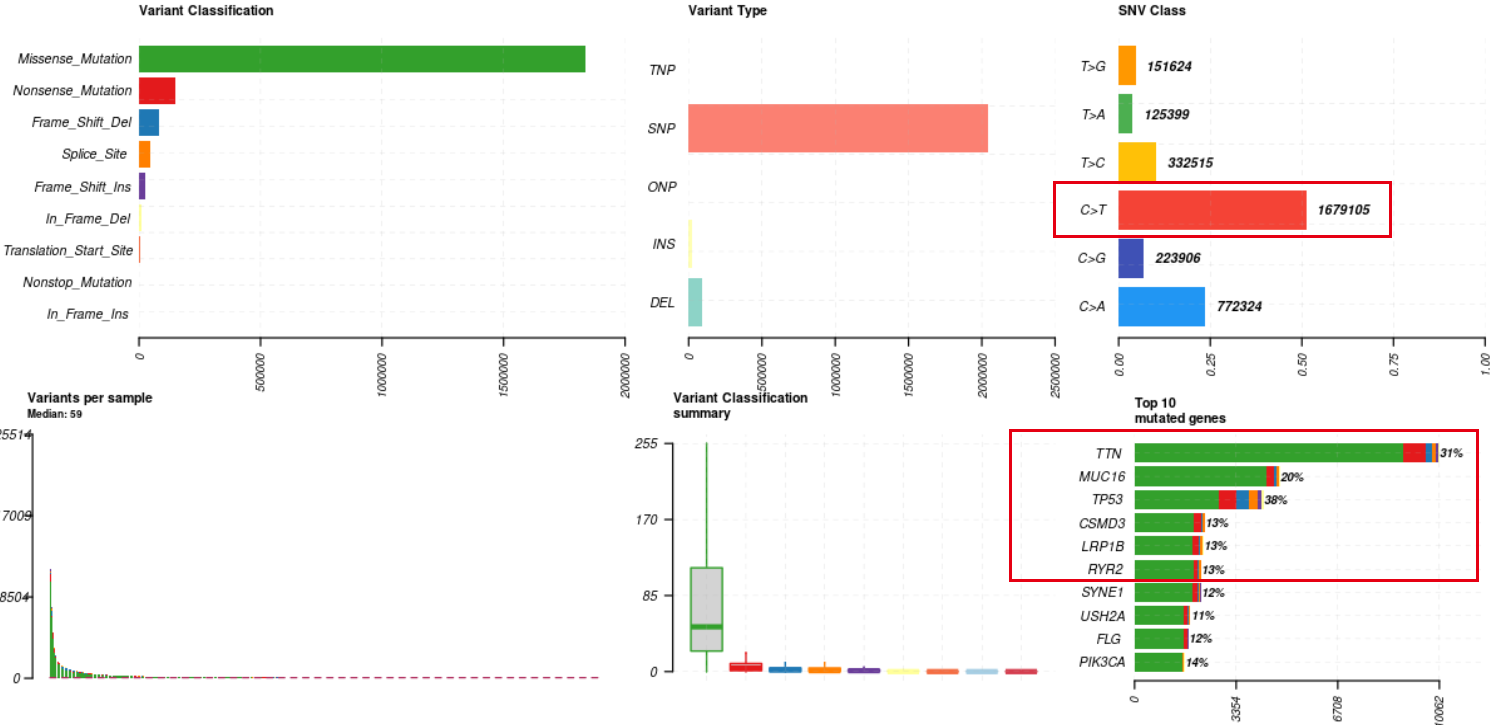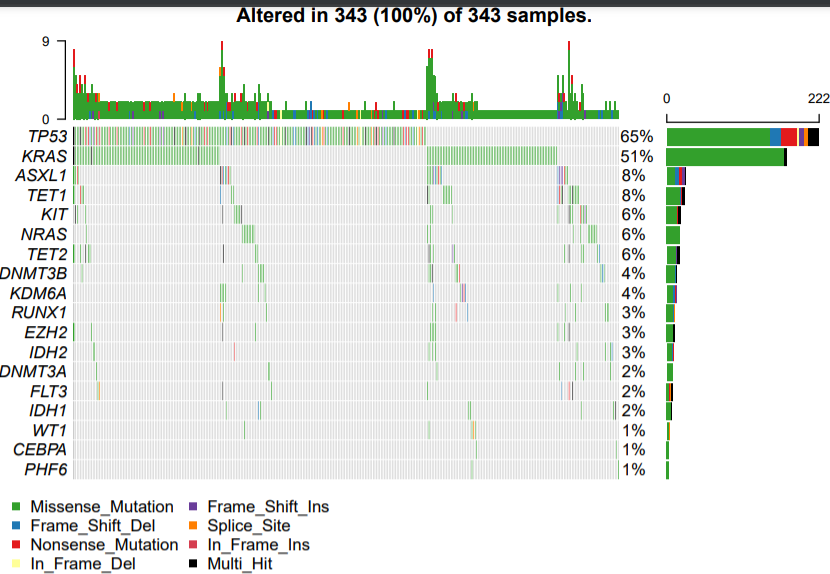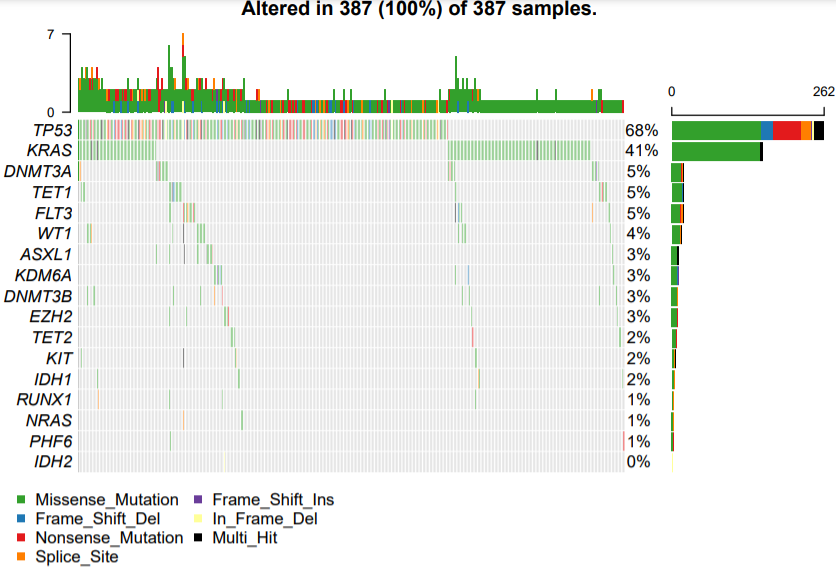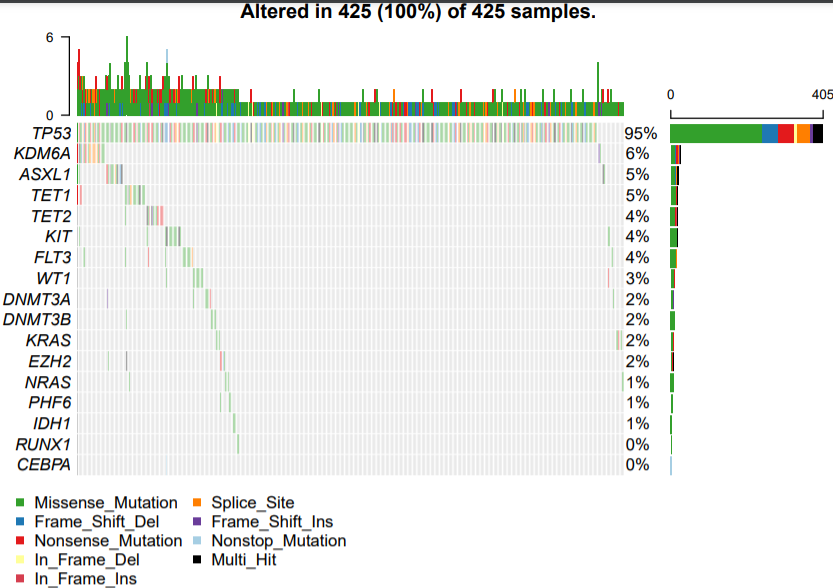# 指定病人的指定基因的突变全景瀑布图

• MuTect2 Variant Aggregation and Masking (n=986) GDC Hub

``````rm(list = ls())
require(maftools)
laml
save(laml,file = 'mc3.Rdata')

rm(list = ls())
require(maftools)

plotmafSummary(maf = laml,
rmOutlier = TRUE, addStat = 'median',
dashboard = TRUE, titvRaw = FALSE)
````````````rm(list = ls())
require(maftools)
options(stringsAsFactors = F)
library(data.table)

cg_tmp=tmp[tmp\$Hugo_Symbol %in% cg,]
cg_cp_tmp=cg_tmp[substring(cg_tmp\$Tumor_Sample_Barcode,1,15) %in% cp,]

oncoplot(maf = laml, top = 10) #高频突变的前10个基因，如下图所示
oncoplot(maf = laml,top = 10,draw_titv = TRUE) #在oncoplot中叠加titv图
``````

### 首先过滤基因

``````rm(list = ls())
require(maftools)

Genes = c(
"TP53",
"WT1",
"PHF6",
"DNMT3A",
"DNMT3B",
"TET1",
"TET2",
"IDH1",
"IDH2",
"FLT3",
"KIT",
"KRAS",
"NRAS",
"RUNX1",
"CEBPA",
"ASXL1",
"EZH2",
"KDM6A"
)

tmp=laml@data
cg_tmp=tmp[tmp\$Hugo_Symbol %in% Genes,]
``````

### 批量进行每个癌症内部绘图

``````library(readxl)
phe=as.data.frame(phe)

bcr_patient_barcode type
1 TCGA-OR-A5J1 ACC
2 TCGA-OR-A5J2 ACC
3 TCGA-OR-A5J3 ACC
4 TCGA-OR-A5J4 ACC
5 TCGA-OR-A5J5 ACC
6 TCGA-OR-A5J6 ACC
``````

``````
table(phe\$type)
cp_list=split(phe\$bcr_patient_barcode,phe\$type)

lapply(1:length(cp_list), function(i){
cp=cp_list[[i]]
pro=names(cp_list)[i]
cg_cp_tmp=cg_tmp[substring(cg_tmp\$Tumor_Sample_Barcode,1,12) %in% cp,]
pdf(paste0('choose-genes-in-',pro,'.pdf'),height = 5)
oncoplot(maf = laml , removeNonMutated =F) #高频突变的前10个基因
dev.off()
})
``````• 掌握肿瘤变异calling与注释的基本方法，对于影响变异的特殊因素及其解决方案有一定的认识。

• 掌握肿瘤免疫NGS相关分析，学会肿瘤免疫治疗的队列景观分析的研究方法。

• 掌握肿瘤克隆与进化的研究方法与手段，解析肿瘤队列挖掘关键基因，结合数据库进行解读以发表高水平研究的套路与方法。

• 适当拓展肿瘤NGS多组学研究的方法与手段。

• 最终可实现初步具备开展肿瘤变异研究的相关课题或者搭建与开发肿瘤相关应用产品的能力。